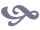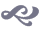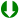#Homework NotesI will begin posting general comments regarding the homework answers and grades here. Please take a look at the comments even if you did perfectly on the homework.

## Homework 8

### Problem 1

The point emphasis of this problem was placed on correctly classifying the language, and less on properly proving your claim is true (especially since problems 2, 3, and 4 deal with providing the proper proofs). However, you need to be sure you understand how to properly prove a language is decidable, acceptable, or neither acceptble nor co-acceptable.

It is also important to realize the relationships between different language classes. For example, a proof that a language is undecidable does not also prove that language is not acceptable. The acceptance problem for Turing machines is a good example, as it is both undecidable and acceptable.

• If you know a language is acceptable, it may or may not be decidable.
• If you know a language is undecidable, it may or may not be acceptable.
• If you know a language is not acceptable and undecidable, it may or may not be co-acceptable.

So in these cases, you need to do further investigation of the language to determine its tightest classification.

### Problem 2, 3, and 4

When providing a reduction proof, you need to do so formally at this point in the course. This means using mapping reducibility. Look in the book or discussion notes for more on how to do these proofs (or visit me during office hours).

### Problem 3

If you defined L as any Turing recognizable language, we want to show that L reduces to ATM. Since L is Turing recognizable, we know there is some Turing machine M which recognizes L.

However, we do not know what form of input M accepts. Many people incorrectly assumed the input was <M, w>. For example, L could be:

• L = { <M> | M is a Turing machine }
• L = { w in { 0, 1 }* | w has even length }
• L = { <G> | G is a CFG }
• and so on...
Therefore, we can not make any assumptions about the input of M.

### Problem 5

I did not grade this problem. Check the solutions to see if you were correct!

## Homework 7

This homework is worth 45 points. It will be weighted equally with the other homework. The average score was about 29 (64%).

Be sure you understand how to do these (and past) problems. You will need this knowledge for the final.Homework 7 Grading Notes [ pdf ]

### Problem 1

You can download a JFLAP file of this unrestricted grammar and play around with different inputs to convince yourself it is correct.

Warning: If you decide to test grammars with several rules or long inputs, it may take a very long time for JFLAP to compute if that word is accepted or rejected by your grammar. For example, for answers with more than 5 rules, the string aaaaabbbbbccccc typically took several minutes on my 2.8GHz computer (with 1GB of RAM).

## Homework 6

This homework is worth 50 points. It will be weighted equally with the other homework. The average score was about 30 (60%).Homework 6 Grading Notes [ pdf ]

You may also download JFLAP files for some of the problems:

### Problem 3

Here is what the PDA should look like. You can download a JFLAP file of this PDA and play around with different inputs to convince yourself it is correct.

### Problem 5

Here is what the TM should look like. You can download a JFLAP file of this PDA and play around with different inputs to convince yourself it is correct.

### Problem 2

• You had to fully and formally specify how to build a DFA, NFA, or regular expression from the regular grammar.

• The average score was around ?? out of 10 points.

## Homework 5

This homework is worth 60 points. However, it will be weighted equally with the other homework. The average score was about 40.

### Problem 1

• Please look at the homework solutions for how to solve this problem using the pumping lemma. It is important you understand the intuition behind this.

• You can reuse decision procedures and algorithms that we have already gone over (in lecture, discussion, or in book). However, if we have not covered a method for doing something you need to provide the algorithm yourself.

Therefore you cannot just say look for a cycle... you must also provide a method for finding cycles. We have discussed how to determine if a state is reachable, which can be used to find cycles. However that algorithm alone does not find cycles, it just tests the reachability of states.

• Be specific when reusing previously covered algorithms and decision procedures. For example, we do not give an algorithm for converting a regular expression to a DFA. We give an algorithm for converting the regular expression to an NFA, and an algorithm for converting an NFA to a DFA. (This requirement has not changed since the first decision procedures you were asked to give!)

• The average score was around 6 out of 10 points.

### Problem 2

• I did not grade part (c), but be sure to check the homework solutions to make sure you got it correct.

• Let L = { ai bj ck | i < j and i < k } and L' = { ai bj ck | 0 <= i <= j <= k }. Notice that L != L' (try string abbbcc)! The language L' is given in the book, but L is not. Therefore if you used L for part (b) you needed to provide a proof that it is not context free.

• The average score was almost 9 out of 10 points.

### Problem 3

• There was an error on the homework solutions. The correct solution is given on the discussion boards.

• When grading, I used JFLAP to test your grammars against several test strings. I had a set of test strings for when n > 2m, when n < 2m, and when n = 2m. I suggest you start using JFLAP if you have not already. You could do the same before you turnin your homework, and be certain you have the correct grammar!• You could have done this by splitting this problem into two cases. If L1 is the case when n < 2m and L2 is the case when n > 2m, then our language L is the union of L1 and L2.

When n < 2m, every time we add a "b" symbol we may add 0, 1, or 2 "a" symbols. When n > 2m, every time we add a "b" symbol we must add 2 "a" symbols. The resulting grammar is on the discussion board.

• The average score was almost 5 out of 10 points.

### Problem 4

• It was important to include in your description for part (a) that the number of a symbols was not only even, but also greater than 0 (or greater than or equal to 2).

• The average score was about 8.5 out of 10 points.

### Problem 5

• Finding a proper word was key to part (a). Here is some intuition for choosing a good word for this problem. You can probably guess that x will have to contain the # symbol (see the solutions for reasoning). We know that vxy <= p. Therefore we would like to limit the possibilities for v and y. We can do this by surrounding the # symbol with unary strings of length p.

This gives us something that looks like 0p # 1p. Of course, the string x might look something like # or 0 # 1, but we know that v can only consist of 0s and y can only consist of 1s. From here, we need to fix our string so that it is actually a string in our language.

• There were three cases you needed to have for part (a):

1. When vxy did not contain a #
2. When vxy does contain a #
1. When v or y contains the #
2. When x contains the #
• The average score was about 12 out of 20 points.

### Problem 6

• I did not grade this problem, but you can check the homework solutions to see if you got it correct.